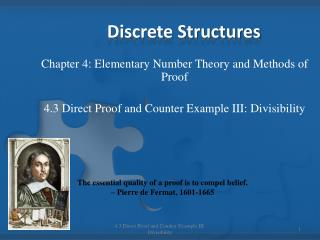Download PresentationDiscrete Structures

Loading in 2 Seconds...

# Discrete Structures - PowerPoint PPT Presentation

Discrete Structures. Chapter 4: Elementary Number Theory and Methods of Proof 4.3 Direct Proof and Counter Example III: Divisibility. The essential quality of a proof is to compel belief. – Pierre de Fermat, 1601-1665. Definitions. If n and d are integers and d  0 thenI am the owner, or an agent authorized to act on behalf of the owner, of the copyrighted work described.
Download Presentation## Discrete Structures

An Image/Link below is provided (as is) to download presentation

Download Policy: Content on the Website is provided to you AS IS for your information and personal use and may not be sold / licensed / shared on other websites without getting consent from its author.While downloading, if for some reason you are not able to download a presentation, the publisher may have deleted the file from their server.

- - - - - - - - - - - - - - - - - - - - - - - - - - E N D - - - - - - - - - - - - - - - - - - - - - - - - - -
Presentation Transcript
1. Discrete Structures Chapter 4: Elementary Number Theory and Methods of Proof 4.3 Direct Proof and Counter Example III: Divisibility The essential quality of a proof is to compel belief. – Pierre de Fermat, 1601-1665 4.3 Direct Proof and Counter Example III: Divisibility

2. Definitions If n and d are integers and d 0 then n is divisible bydiffn equals d times some integer. Instead of “n is divisible by d,” we can say that n is a multiple of d d is a factor of n d is a divisor of n d divides n The notation d | n is read “d divides n.” Symbolically, if n and d are integers and d  0. d | n   an integer k s.t. n = dk. 4.3 Direct Proof and Counter Example III: Divisibility

3. NOTE • Since the negation of an existential statement is universal, it follows that d does not divide niff, for all integers k, n dk, or, in other words, n/d is not an integer. 4.3 Direct Proof and Counter Example III: Divisibility

4. Theorems • Theorem 4.3.1 – A Positive Divisor of a Positive Integer For all integers a and b, if a and b are positive and a divides b, then a b. • Theorem 4.3.2 – Divisors of 1 The only divisors of 1 are a and -1. • Theorem 4.3.3 – Transitivity of Divisibility For all integers a ,b, and c, if a divides b and b divides c, then a divides c. 4.3 Direct Proof and Counter Example III: Divisibility

5. Theorems • Theorem 4.3.4 – Divisibility by a Prime Any integer n > 1 is divisible by a prime number. • Theorem 4.3.5 – Unique Factorization of Integers Theorem (Fundamental Theorem of Arithmetic) 4.3 Direct Proof and Counter Example III: Divisibility

6. Definition 4.3 Direct Proof and Counter Example III: Divisibility

7. Example – pg. 178 # 12 • Give a reason for your answer. Assume that all variable represent integers. 4.3 Direct Proof and Counter Example III: Divisibility

8. Example – pg. 178 # 15 • Prove the statement directly from the definition of divisibility. 4.3 Direct Proof and Counter Example III: Divisibility

9. Example – pg. 178 # 27 • Determine whether the statement is true or false. Prove the statement directly from the definitions if it is true, and give a counterexample if it is false. 4.3 Direct Proof and Counter Example III: Divisibility

10. Example – pg. 178 # 28 • Determine whether the statement is true or false. Prove the statement directly from the definitions if it is true, and give a counterexample if it is false. 4.3 Direct Proof and Counter Example III: Divisibility

11. Example – pg. 178 # 35 • Two athletes run a circular track at a steady pace so that the first completes one round in 8 minutes and the second in 10 minutes. If they both start from the same spot at 4 pm, when will be the first they return to the start together. 4.3 Direct Proof and Counter Example III: Divisibility

12. Example – pg. 178 # 37 • Use the unique factorization theorem to write the following integers in standard factored form. • b. 5,733 • c. 3,675 4.3 Direct Proof and Counter Example III: Divisibility